Definition: quadrilateral from The Penguin Dictionary of Science

A four-sided ➤polygon.

from Teach Yourself: Mathematics: A Complete Introduction

A quadrilateral is a shape with four straight sides and four angles. You can investigate the sum of the angles of a quadrilateral in a way similar to the one you used for a triangle.

Draw a quadrilateral and label its angles a, b, c and d (Figure 4.17).Tear off the corners and fit the angles together at a point (Figure 4.18). From Chapter 2, the angles at a point add up to 360°. This suggests that a + b + c + d = 360° and that the angle sum of a quadrilateral is 360°.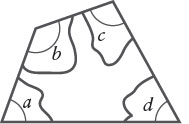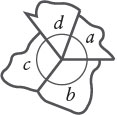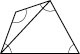To prove this, draw a diagonal of a quadrilateral, dividing it into two triangles (see Figure 4.19). The angle sum of each triangle is 180°, so the angle sum of a quadrilateral is 360°.

A square is a quadrilateral with four equal sides and four right angles (Figure 4.20). A square has 4 lines of symmetry (Figure 4.21), and rotational symmetry of order 4.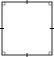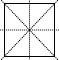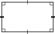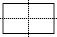A rectangle has four right angles and its opposite sides are equal in length (Figure 4.22). A rectangle has 2 lines of symmetry (Figure 4.23), and rotational symmetry of order 2.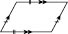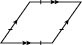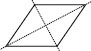A parallelogram has opposite sides which are both parallel and equal in length (see Figure 4.24). A parallelogram has no lines of symmetry, and rotational symmetry of order 2.

A rhombus (Figure 4.25) is a parallelogram with equal sides. It has 2 lines of symmetry (Figure 4.26), and rotational symmetry of order 2.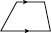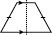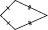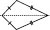A trapezium (Figure 4.27) has one pair of parallel sides. It generally has no lines of symmetry and has rotational symmetry of order 1. An isosceles trapezium (Figure 4.28), in which the non-parallel sides are equal in length, has one line of symmetry.

A kite (Figure 4.29) has two pairs of adjacent sides with equal lengths, like two isosceles triangles with their bases joined. A kite has 1 line of symmetry (Figure 4.30), and rotational symmetry of order 1.© Hodder Education, an Hachette UK Company 2013

Related Articles

Merriam-Webster's Collegiate(R) Dictionary

pronunciation (1650) : a polygon of four sides